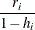# The REG Procedure

### Model Fit and Diagnostic Statistics

This section gathers the formulas for the statistics available in the MODEL , PLOT , and OUTPUT statements. The model to be fit is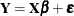, and the parameter estimate is denoted by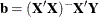. The subscript i denotes values for the ith observation, the parenthetical subscript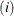means that the statistic is computed by using all observations except the ith observation, and the subscript jj indicates the jth diagonal matrix entry. The ALPHA= option in the PROC REG or MODEL statement is used to set thevalue for the t statistics.

Table 97.7 contains the summary statistics for assessing the fit of the model.

Table 97.7: Formulas and Definitions for Model Fit Summary Statistics

Model Option or Statistic

Definition or Formula

n

the number of observations

p

the number of parameters including the intercept

i

1 if there is an intercept, 0 otherwise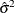the estimate of pure error variance from the SIGMA=
option or from fitting the full model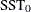the uncorrected total sum of squares for the dependent
variable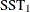the total sum of squares corrected for the mean for the
dependent variable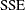the error sum of squares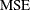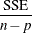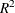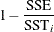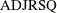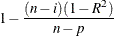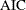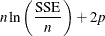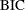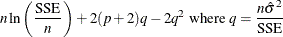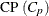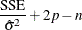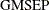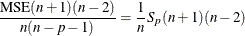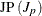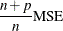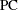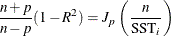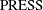the sum of squares of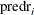(see Table 97.8)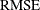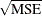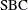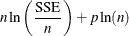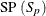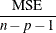Table 97.8 contains the diagnostic statistics and their formulas; these formulas and further information can be found in Chapter 4: Introduction to Regression Procedures, and in the section Influence Statistics. Each statistic is computed for each observation.

Table 97.8: Formulas and Definitions for Diagnostic Statistics

MODEL Option or Statistic

Formula

PRED (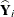)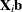RES (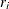)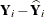H (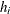)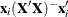STDP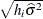STDI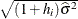STDR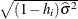LCL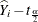STDI

LCLMSTDP

UCL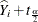STDI

UCLMSTDP

STUDENT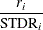RSTUDENT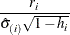COOKD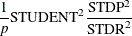COVRATIO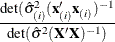DFFITS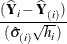DFBETAS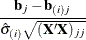PRESS()